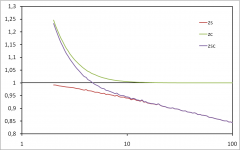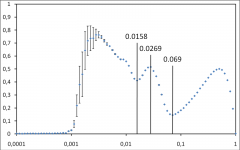Tag Archives: random graph

Plots

Bias in generated random graphs> By Fabien Viger and Matthieu Latapy One of the main approaches for modelling complex networks is to sample a random graph with prescribed degree distribution; in other words, one chooses a graph uniformly at random (i.e. all graphs have the same probability to be chosen) among all the graphs with a given number N […]

Posted in Plots | Also tagged |
Plots

Random graph exploration with shortest paths> Matthieu Latapy and Jean-Loup Guillaume A random graph of n nodes may be constructed by adding each possible link with a given probability p. The obtained graph then has density p. One may roughly model traceroute measurements of the internet topology from one monitor as the collection of all shortest paths from this monitor […]

Posted in Plots | Also tagged , |